Excel 2013 contains powerful tools to save you hours of calculations. The Excel solver function for example, can help you work out what optimum levels of production or sales will be based on various objectives and constraints. This tutorial will show you how solver works and how you can use it to determine how many units to sell in different branches of an organization.

If you are familiar with Excel and want to take your skills to the next level, enroll in Learn Microsoft Excel 2013 – Advanced now and join over four thousand students who are learning to use Excel like a pro. This course offers over 52 lessons and 12 hours of video content to teach you how to take advantage of advanced Excel functions. You will learn how to work with dates and times, how to calculate depreciation, how to insert and format tables and how to work with Pivot tables and charts.

For this tutorial we will assume you work for a computer company that has branches in California, Texas and Ohio. The company produces PC’s that they sell at each of the different branches at different prices based on the area because the PC’s in California are slightly faster and therefore people pay slightly more for the PC than in Ohio, for example. We know the inputs for each PC and we also how many units of input we have. What we need to work out is how many PC’s we should make in each area to maximize the company’s profit. If we manually calculated the figures, it could take hours to work out the most profitable combination, but with Excel Solver, Excel does the work for you.

Here is the data we have to begin with:We know the sales price of the PC’s in each area – shown in the PC profit column. We also know what the inputs are for each area – seen under the Inputs for each PC. We also know how many inputs we have on hand.

Our objective is to maximize profit so cell E5 will be our objective cell and it will show us what profit we will make if we sell the optimal number of computers in each branch.

Our variables for this problem are the unit sales for each area. The sales are represented by B4; C4 and D4. We need Excel to work out what the optimal sales for each branch will be based on the inputs we have to maximize our profit.

The components we have represent the constraints of the problem. We can’t make more units than we have in stock.

So we have all of the components we need to get Excel to use the solver to work out the best combination of sales for each branch.

## Add the Formulas for the Cells

Add the formula for the Total Profit: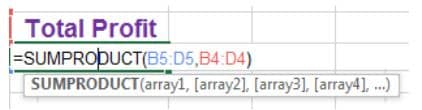We will use the SUMPRODUCT formula to calculate the profit. Profit is calculated by multiplying the units sold times the unit price for each area. We can use the same SUMPRODUCT formula to calculate how many inputs are used for each type of component: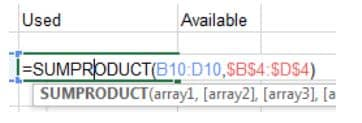Our worksheet now contains all of the formulas we need to calculate the optimal level of sales per branch.

For lessons on the advanced functions available in Excel, enroll in Microsoft Excel 2013 Advanced Online Excel Training Course now and join over twenty three thousand students who are learning to harness the power of the advanced features and functions in Excel. This course offers over 128 lectures and over 10 hours of content designed to take your Excel skills to the next level. You will gain a solid understanding of most of the powerful features available in Excel and after the course you will feel comfortable about using Excel in any commercial environment.

## Using Excel Solver

Click the Data tab and then click solver. Solver opens a new window to allow you to add your constraints and objectives: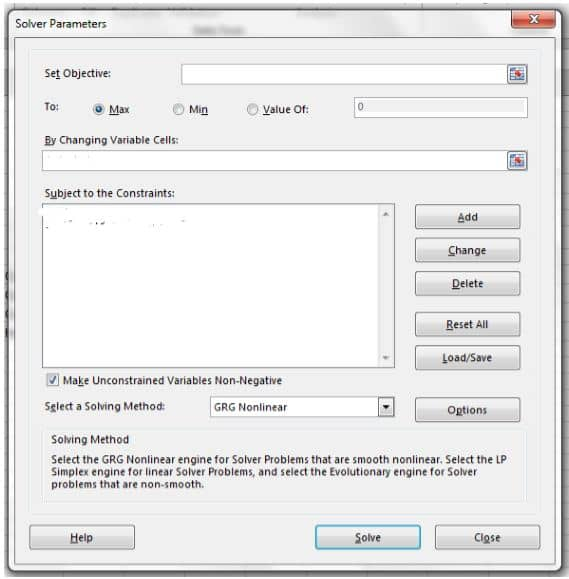Click the “Set Objective” field and then select the Profit cell – this will set the objective as the maximum sales that Excel can calculate based on the variables and constraints. We will leave the “To:” value at Max since we want to achieve maximum profit.

The variables for our calculation are the sales per branch. What we are trying to work out is the best combination of sales per branch to maximize profit so the variables are sales per area. So select cell B4:D4 as the variable range.

Now we need to add the constraints to our solver. The constraints involved in this example is the fact that we cannot produce more computers than we have components for and we also can’t produce a portion of a computer – we have to produce one whole computer at a time.

To enter the first constraint, we therefore need to say that the components used must not be greater than the ones we have on hand. So click “Add” to add a constraint. Then select E10:E13 – this will be the number of units used to produce the PC’s and then select the “<=” and then select F10:F13. In other words we are telling Excel that the number of units used must be less than or equal to the units we have on hand. The constraint will look like this: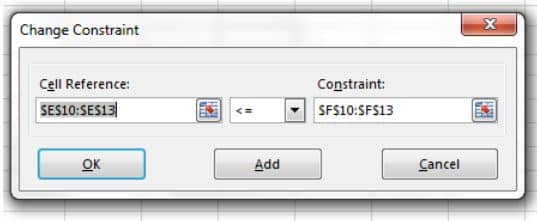Now we must add a constraint to ensure that Excel knows it can’t produce less than one computer. The constraint will therefore be unit sales must be greater than or equal to one.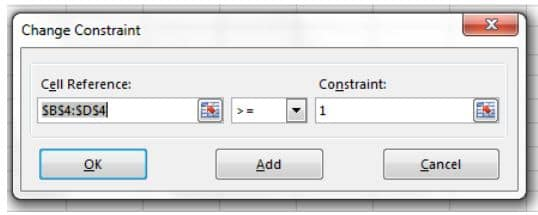Now that we have all the components, click solve.

The answer appears as follows: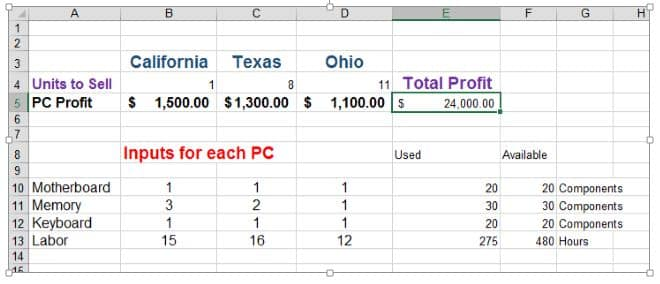It would have taken hours and many calculations to work out the combination, but Excel’s solver 2013 has turned the problem into a solution in no time at all.

Become an Excel master. Check out Microsoft Excel 2013 Beginners/Intermediate Training and go from zero to Excel hero. This course offers over 58 lectures and 14 hours of content designed to take you from beginner level in Excel to Excel master. The course will introduce you to the basics of Excel and then build on your knowledge until you are able to master advanced Excel topics like working with Pivot charts, analyzing data and financial analysis.

Page Last Updated: June 2014

### Top courses in Excel

Microsoft Excel - Excel from Beginner to Advanced
Kyle Pew, Office Newb
4.7 (331,349)
Bestseller
Microsoft Excel - Data Visualization, Excel Charts & Graphs
Maven Analytics, Chris Dutton
4.6 (33,433)
Bestseller
Microsoft Excel - Data Analysis with Excel Pivot Tables
Maven Analytics, Chris Dutton
4.7 (42,318)
Bestseller
Microsoft Excel - Excel from Beginner to Advanced 2022
Warrick Klimaytys
4.7 (1,022)
Bestseller
Microsoft Excel Pro Tips: Go from Beginner to Advanced Excel
Maven Analytics, Chris Dutton
4.6 (3,695)
Bestseller
Microsoft Excel for Construction Management
James Gedney-Higham
4.5 (349)
Bestseller

## Empower your team. Lead the industry.

Get a subscription to a library of online courses and digital learning tools for your organization with Udemy Business.